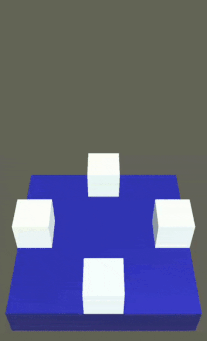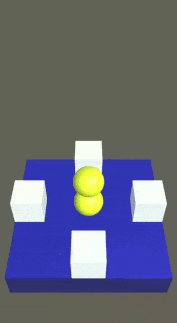# How to detect single tap

Hello people. Im currently working on my first game. In game, player can spawn objects by single tapping on the screen.

Here is the basic code:

``````private void Update()
{
if (Input.touchCount > 0 && Input.GetTouch(0).phase == TouchPhase.Began)
{
//Code of placing object
}
}
``````

And here is a simple demo of it:But also player can rotate the camera by swiping left or right across the screen.

Camera rotation code:

``````public class CameraRotator : MonoBehaviour //Script attached to the main camera
{
private Vector3 firstpoint;
private Vector3 secondpoint;
private float xAngle = 0.0f;
private float xAngTemp = 0.0f;
private void Start()
{
xAngle = 0.0f;
transform.rotation = Quaternion.Euler(0, xAngle, 0.0f);
}
private void Update()
{
if (Input.touchCount > 0)
{
if (Input.GetTouch(0).phase == TouchPhase.Began)
{
firstpoint = Input.GetTouch(0).position;
xAngTemp = xAngle;
}

if (Input.GetTouch(0).phase == TouchPhase.Moved)
{
secondpoint = Input.GetTouch(0).position;
xAngle = xAngTemp + (secondpoint.x - firstpoint.x) * 180.0f / Screen.width;
transform.rotation = Quaternion.Euler(0, xAngle, 0.0f);
}
}
}
}
``````

But there is a problem that the object also spawns at the beginning of rotation.

Here is a simple demo of it:How can I separate these inputs from each other, so that the objects does not spawn when the camera rotates? How to differentiate single tap and swipe?

Hello,Rammart
You may try to compare the firstpoint and the secondpoint variable. If they are equal that means input is a tap if they are not equal that menas input is a swipe.

Also you should replace your TouchPhase.Began with TouchPhase.Ended on line 3 (the script that spawns objects).

Try to change your Update function in your spawning script to that

`````` private void Update()
{
//Let the computer find the object script is attached to so you don't have to do it.
CameraRotator cameraRotator = FindObjectOfType<CameraRotator>();
if (Input.touchCount > 0 && Input.GetTouch(0).phase == TouchPhase.Ended)
{
if(firstpoint == secondpoint)
{
//Code of placing object
}
}
}
``````

Feel free to ask if you have any questions.
Hope this helps.